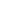New article submisson system
Submit an article in minutesOriginal research

# An Effective Approach to Detect Liver Disorder using KNN Algorithm in Comparison with Decision Tree Algorithm to Measure Accuracy

## Abstract

Aim: An effective approach to detect liver disorder using KNN algorithm in comparison with decision tree algorithm to measure accuracy. Methods and Materials: For identification of effective approaches to detect liver disorder, the KNN algorithm is used comparatively with the decision tree algorithm which is an existing algorithm. The number of samples used in each group is 20. Result: It is discovered that the K- nearest neighbor (KNN) algorithm gives better mean accuracy than the decision tree algorithm. Each sample has a distinct level of accuracy, with KNN algorithm having a maximum accuracy value of 95.75%, which is higher than the decision tree algorithm 93.40%. The statistical results show that the KNN algorithm and decision tree algorithms have distinct statistical significance levels of p=0.001, i.e., p<0.05 (independent sample T-test). Conclusion: On the basis of a liver disease investigation, it is obvious that the K- nearest neighbor algorithm has a higher mean accuracy value than the decision tree method. The KNN algorithm has a 94.30% mean accuracy rating, whereas decision Tree has a 91.36% rating.

## Imprint

M. Mohammed Zaheer, P. Nirmala. An Effective Approach to Detect Liver Disorder using KNN Algorithm in Comparison with Decision Tree Algorithm to Measure Accuracy. Cardiometry; Issue 25; December 2022; p.1038-1046; DOI: 10.18137/cardiometry.2022.25.10381046; Available from: https://www.cardiometry.net/issues/no25-december-2022/knn-algorithm-comparison-decision-tree

## Keywords

K- nearest neighbor algorithm,  Decision Tree algorithm,  Liver disorder,  Artificial intelligence,  Innovative liver disorder detectionCurrent issueCardiometry's libraryFounders of Cardiometry